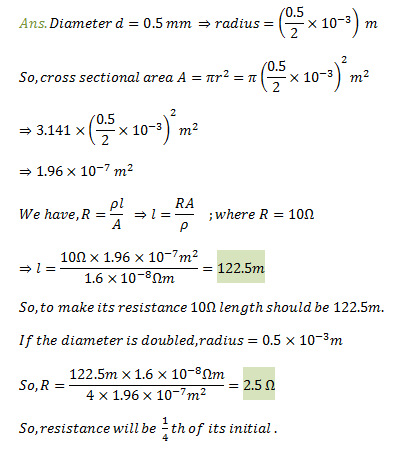3 Tutor System
Starting just at 265/hour

# A copper wire has diameter 0.5 mm and resistivity of $$1.6 × 10^-8 \Omega m.$$ What will be the length of this wire to make its resistance 10 $$\Omega$$? How much does the resistance change if the diameter is doubled?

Area of cross-section of the wire, A =$$\pi$$ (d/2) 2 Diameter= 0.5 mm = 0.0005 m Resistance, R = 10 $$\Omega$$ We know that